# Heat Distribution in Circular Cylindrical Rod

This example shows how to simplify a 3-D axisymmetric thermal problem to a 2-D problem using the symmetry around the axis of rotation of the body.

This example analyzes heat transfer in a rod with a circular cross section. There is a heat source at the bottom of the rod and a fixed temperature at the top. The outer surface of the rod exchanges heat with the environment because of convection. In addition, the rod itself generates heat because of radioactive decay. The goal is to find the temperature in the rod as a function of time.

The model geometry, material properties, and boundary conditions must all be symmetric about the axis of rotation. The toolbox assumes that the axis of rotation is the vertical axis passing through r = 0.

First, compute the steady-state solution. If the final time in the transient analysis is sufficiently large, the transient solution at the final time must be close to the steady state solution. By comparing these two results, you can check the accuracy of the transient analysis.

Create a steady-state thermal model for solving an axisymmetric problem.

`thermalModelS = createpde('thermal','steadystate-axisymmetric');`

The 2-D model is a rectangular strip whose x-dimension extends from the axis of symmetry to the outer surface and y-dimension extends over the actual length of the rod (from `-`1.5 m to 1.5 m). Create the geometry by specifying the coordinates of its four corners.

`g = decsg([3 4 0 0 .2 .2 -1.5 1.5 1.5 -1.5]');`

Include the geometry in the model.

`geometryFromEdges(thermalModelS,g);`

Plot the geometry with the edge labels.

```figure pdegplot(thermalModelS,'EdgeLabels','on') axis equal```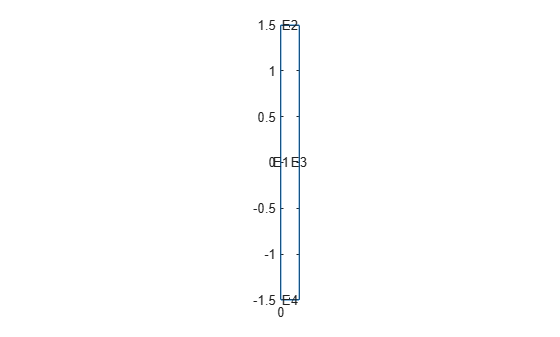The rod is composed of a material with these thermal properties.

```k = 40; % Thermal conductivity, W/(m*C) rho = 7800; % Density, kg/m^3 cp = 500; % Specific heat, W*s/(kg*C) q = 20000; % Heat source, W/m^3```

For a steady-state analysis, specify the thermal conductivity of the material.

`thermalProperties(thermalModelS,'ThermalConductivity',k);`

Specify the internal heat source.

`internalHeatSource(thermalModelS,q);`

Define the boundary conditions. There is no heat transferred in the direction normal to the axis of symmetry (edge 1). You do not need to change the default boundary condition for this edge. Edge 2 is kept at a constant temperature T = 100 °C.

`thermalBC(thermalModelS,'Edge',2,'Temperature',100);`

Specify the convection boundary condition on the outer boundary (edge 3). The surrounding temperature at the outer boundary is 100 °C, and the heat transfer coefficient is $50\text{\hspace{0.17em}}\mathrm{W}/\left(\mathrm{m}{\cdot }^{\circ }\mathrm{C}\right)$.

```thermalBC(thermalModelS,'Edge',3,... 'ConvectionCoefficient',50,... 'AmbientTemperature',100);```

The heat flux at the bottom of the rod (edge 4) is $5000\text{\hspace{0.17em}}\mathrm{W}/{\mathrm{m}}^{2}$.

`thermalBC(thermalModelS,'Edge',4,'HeatFlux',5000);`

Generate the mesh.

```msh = generateMesh(thermalModelS); figure pdeplot(thermalModelS) axis equal```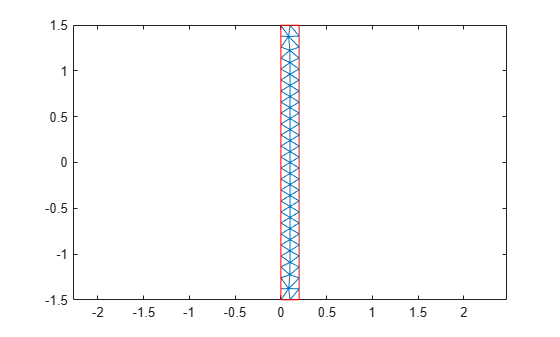Solve the model and plot the result.

```result = solve(thermalModelS); T = result.Temperature; figure pdeplot(thermalModelS,'XYData',T,'Contour','on') axis equal title 'Steady-State Temperature'```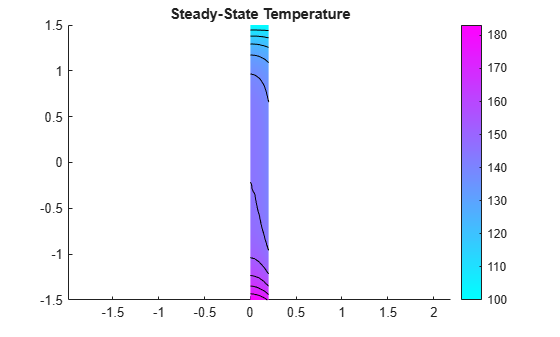### Transient Solution

Create a transient thermal model for solving an axisymmetric problem.

`thermalModelT = createpde('thermal','transient-axisymmetric');`

Use the same geometry and mesh as for the steady-state analysis.

```g = decsg([3 4 0 0 .2 .2 -1.5 1.5 1.5 -1.5]'); geometryFromEdges(thermalModelT,g); thermalModelT.Mesh = msh;```

Specify the thermal conductivity, mass density, and specific heat of the material.

```thermalProperties(thermalModelT,'ThermalConductivity',k,... 'MassDensity',rho,... 'SpecificHeat',cp);```

Specify the internal heat source and boundary conditions.

```internalHeatSource(thermalModelT,q); thermalBC(thermalModelT,'Edge',2,'Temperature',100); thermalBC(thermalModelT,'Edge',3,... 'ConvectionCoefficient',50,... 'AmbientTemperature',100); thermalBC(thermalModelT,'Edge',4,'HeatFlux',5000);```

Specify that the Initial temperature in the rod is 0 °C.

`thermalIC(thermalModelT,0);`

Compute the transient solution for solution times from t = 0 to t = 50000 seconds.

```tfinal = 50000; tlist = 0:100:tfinal; result = solve(thermalModelT,tlist);```

Plot the temperature distribution at t = 50000 seconds.

```T = result.Temperature; figure pdeplot(thermalModelT,'XYData',T(:,end),'Contour','on') axis equal title(sprintf('Transient Temperature at Final Time (%g seconds)',tfinal))```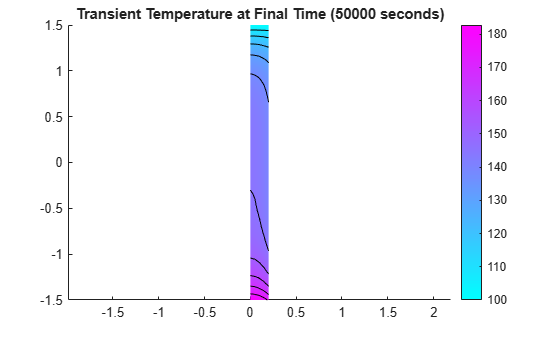Find the temperature at the bottom surface of the rod: first, at the center axis and then on the outer surface.

```Tcenter = interpolateTemperature(result,[0.0;-1.5],1:numel(tlist)); Touter = interpolateTemperature(result,[0.2;-1.5],1:numel(tlist));```

Plot the temperature at the left end of the rod as a function of time. The outer surface of the rod is exposed to the environment with a constant temperature of 100 °C. When the surface temperature of the rod is less than 100 °C, the environment heats the rod. The outer surface is slightly warmer than the inner axis. When the surface temperature is greater than 100 °C, the environment cools the rod. The outer surface becomes cooler than the interior of the rod.

```figure plot(tlist,Tcenter) hold on plot(tlist,Touter,'--') title 'Temperature at the Bottom as a Function of Time' xlabel 'Time, s' ylabel 'Temperature, C' grid on legend('Center Axis','Outer Surface','Location','SouthEast')```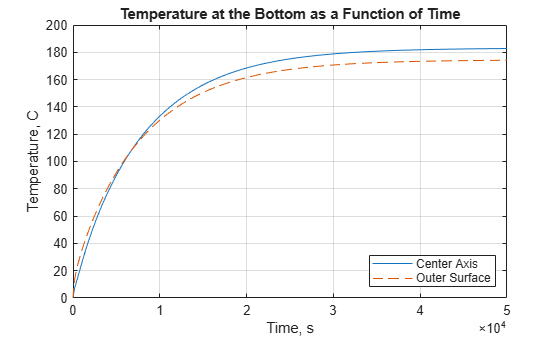## SupportGet trial now